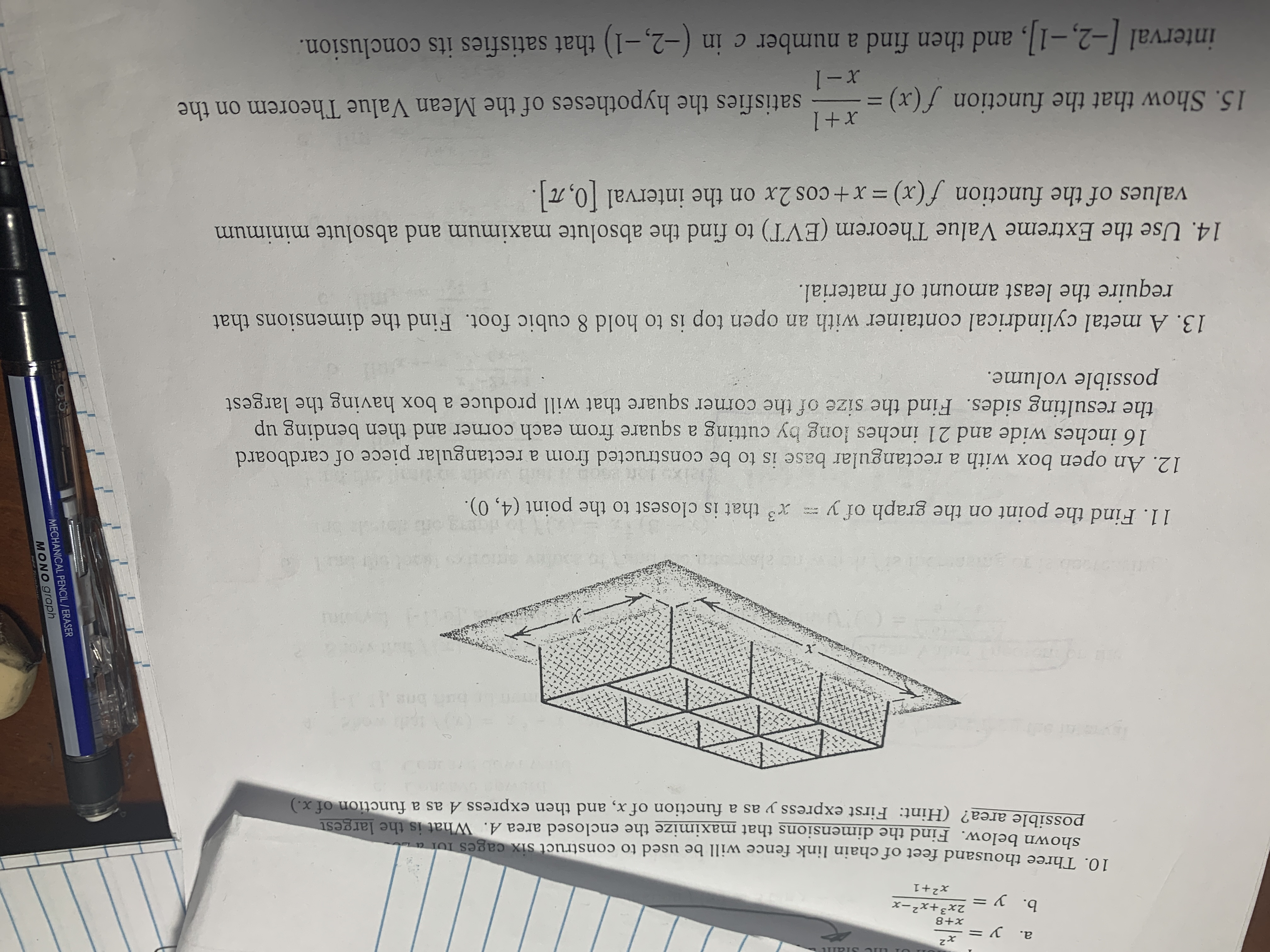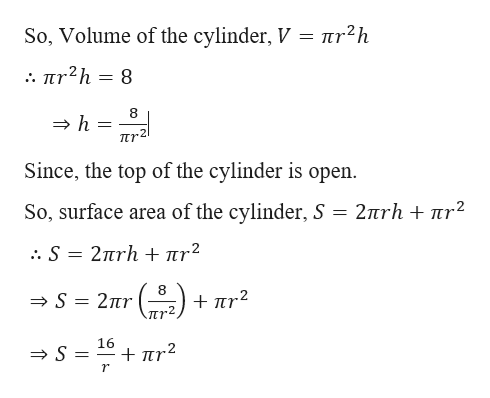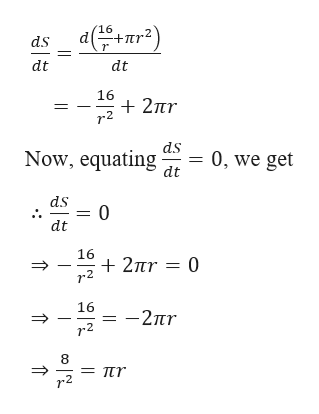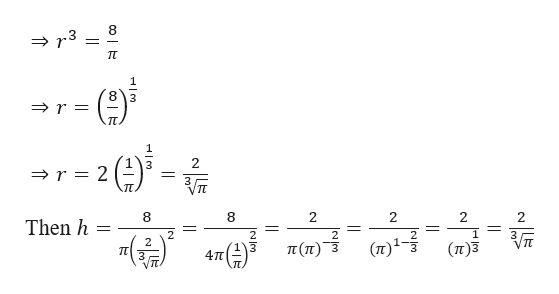# MECHANICAL PENCIL/ERASERx2a. y8+x2x3+x2-xb. y11x2+110. Three thousand feet of chain link fence will be used to construct six cages Ior ashown below. Find the dimensions that maximize the enclosed area A. What is the largestpossible area? (Hint: First express y as a function of x, and then express A as a function of x.)SUG1: sug nen eTuu11. Find the point on the graph of ythat is closest to the point (4, 0).12. An open box with a rectangular base is to be constructed from a rectangular piece of cardboard16 inches wide and 21 inches long by cutting a square from each corner and then bending upthe resulting sides. Find the size of the corner square that will produce a box having the largestpossible volume.13. A metal cylindrical container with an open top is to hold 8 cubic foot. Find the dimensions thatrequire the least amount of material.14. Use the Extreme Value Theorem (EVT) to find the absolute maximum and absolute minimumvalues of the function f(x)=x+ cos 2x on the interval 0,7Tx+115. Show that the function f(x) = satisfies the hypotheses of the Mean Value Theorem on theI-xinterval -2,-1, and then find a number c in (-2,-1) that satisfies its conclusion.

Question
1 views

I need help with number 13. Thankshelp_outlineImage TranscriptioncloseMECHANICAL PENCIL/ERASER x2 a. y 8+x 2x3+x2-x b. y 11 x2+1 10. Three thousand feet of chain link fence will be used to construct six cages Ior a shown below. Find the dimensions that maximize the enclosed area A. What is the largest possible area? (Hint: First express y as a function of x, and then express A as a function of x.) SUG 1: sug nen e Tuu 11. Find the point on the graph of y that is closest to the point (4, 0). 12. An open box with a rectangular base is to be constructed from a rectangular piece of cardboard 16 inches wide and 21 inches long by cutting a square from each corner and then bending up the resulting sides. Find the size of the corner square that will produce a box having the largest possible volume. 13. A metal cylindrical container with an open top is to hold 8 cubic foot. Find the dimensions that require the least amount of material. 14. Use the Extreme Value Theorem (EVT) to find the absolute maximum and absolute minimum values of the function f(x)=x+ cos 2x on the interval 0,7T x+1 15. Show that the function f(x) = satisfies the hypotheses of the Mean Value Theorem on the I-x interval -2,-1, and then find a number c in (-2,-1) that satisfies its conclusion. fullscreen
check_circle

Step 1

Solution to question number 13

Given,

A metal cylindrical container with an open top is to hold 8 cubic foot.help_outlineImage Transcriptioncloseπr?h So, Volume of the cylinder, V . πr2h= 8 8 h = TTr Since, the top of the cylinder is open. So, surface area of the cylinder, S 2πrh + πr? . S=2πrh + πη2 8 S 2nr + πr2 Jur2 16 S = +πr2 r fullscreen
Step 2

Now, differentiating S with respect to t, we gethelp_outlineImage Transcriptionclose16 ds dt dt 16 2ur r2 + 2πr d. Now, equating 0, we get dt ds = 0 dt 16 2r 0 r2 16 -2r r2 8 = nr r2 11 fullscreen
Step 3

Further calcu...help_outlineImage Transcriptionclose8 3 r= 2 3 r = 2 3TT 8 2 2 2 2 Then h 1 2 2 VIT (n)1- π(τ)3 (n)3 TT 4TT fullscreen

### Want to see the full answer?

See Solution

#### Want to see this answer and more?

Solutions are written by subject experts who are available 24/7. Questions are typically answered within 1 hour.*

See Solution
*Response times may vary by subject and question.
Tagged in

### Derivative